• 循环和递归神经网络

千次阅读 2019-07-12 16:06:42
1 循环神经网络 ...前馈神经网络相比，循环神经网络更加符合生物神经网络的结构。循环神经网络已经被广泛应用在语音识别、语言模型以及自然语言生成等任务上。循环神经网络的参数学习可以通过...

1 循环神经网络

循环神经网络（Recurrent Neural Network，RNN）是一类具有短期记忆能力的神经网络。在循环神经网络中，神经元不但可以接受其它神经元的信息，也可以接受自身的信息，形成具有环路的网络结构。和前馈神经网络相比，循环神经网络更加符合生物神经网络的结构。循环神经网络已经被广泛应用在语音识别、语言模型以及自然语言生成等任务上。循环神经网络的参数学习可以通过随时间反向传播算法(BPTT)[Werbos, 1990]来学习。随时间反向传播算法即按照时间的逆 序将错误信息一步步地往前传递。当输入序列比较长时，会存在梯度爆炸和消失问题[Bengio et al., 1994, Hochreiter and Schmidhuber, 1997, Hochreiter et al., 2001]，也称为长程依赖问题。（摘抄：《神经网络与深度学习》）

2 基本循环神经网络结构图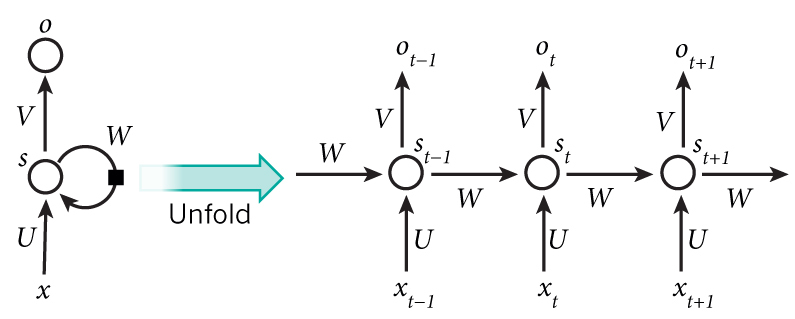如果把上面有W的那个带箭头的圈去掉，它就变成了最普通的全连接神经网络

• x是一个向量，它表示输入层的值；
• s是一个向量，它表示隐藏层的值；
• U是输入层到隐藏层的权重矩阵
• o也是一个向量，它表示输出层的值；
• V是隐藏层到输出层的权重矩阵。那
• 权重矩阵 W就是隐藏层上一次的值作为这一次的输入的权重。因为循环神经网络隐藏层的值s不仅仅取决于当前这次的输入x，还取决于上一次隐藏层的值s。

3 BPTT

随时间反向传播（Backpropagation Through Time，BPTT）算法的主要 思想是通过类似前馈神经网络的错误反向传播算法 [Werbos, 1990] 来进行计算 梯度。 BPTT 算法将循环神经网络看作是一个展开的多层前馈网络，其中“每一 层”对应循环网络中的“每个时刻”。这样，循环神经网络就可以按按照前 馈网络中的反向传播算法进行计算参数梯度。在“展开”的前馈网络中，所有层 的参数是共享的，因此参数的真实梯度是所有“展开层”的参数梯度之和。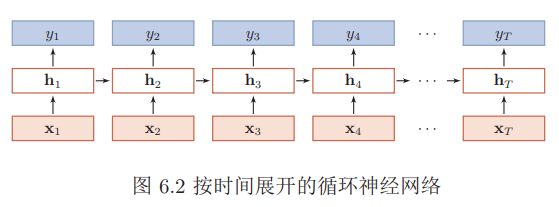BPTT算法是针对循环层的训练算法，它的基本原理和BP算法是一样的，也包含同样的三个步骤：

1. 前向计算每个神经元的输出值；
2. 反向计算每个神经元的误差项值，它是误差函数E对神经元j的加权输入的偏导数；
3. 计算每个权重的梯度。

最后再用随机梯度下降算法更新权重。

4 RNN问题

常见问题：梯度爆炸、梯度消失

梯度爆炸解决方法：

• 权重衰减：通过给参数增加 ℓ1 或 ℓ2 范数的正则化项来限制参数的取值范 围，从而使得γ ≤ 1
• 梯度截断：当梯度的模大于一定 阈值时，就将它截断成为一个较小的数

梯度消失解决方法：

• 合理的初始化权重值。初始化权重，使每个神经元尽可能不要取极大或极小值，以躲开梯度消失的区域。
• 使用relu代替sigmoid和tanh作为激活函数。
• 使用其他结构的RNNs，比如长短时记忆网络（LTSM）和Gated Recurrent Unit（GRU），这是最流行的做法。

5 双向RNN

对于语言模型来说，很多时候光看前面的词是不够的，比如下面这句话：

我的手机坏了，我打算____一部新手机。

可以想象，如果我们只看横线前面的词，手机坏了，那么我是打算修一修？换一部新的？还是大哭一场？这些都是无法确定的。但如果我们也看到了横线后面的词是『一部新手机』，那么，横线上的词填『买』的概率就大得多了。

在上面的句子中，可以看到，一个时刻的输出不但和过去时刻的信息有关，也和后续时刻的信息有关。比如给定一个句子，其中一个词的词性由它的上下文决定，即包含 左右两边的信息。因此，在这些任务中，我们可以增加一个按照时间的逆序来传 递信息的网络层，来增强网络的能力。 双向循环神经网络（Bidirectional Recurrent Neural Network，Bi-RNN）由 两层循环神经网络组成，它们的输入相同，只是信息传递的方向不同。假设第1层按时间顺序，第2层按时间逆序，在时刻t时的隐状态定义为6 递归神经网络

有时候把句子看做是词的序列是不够的，比如下面这句话『两个外语学院的学生』：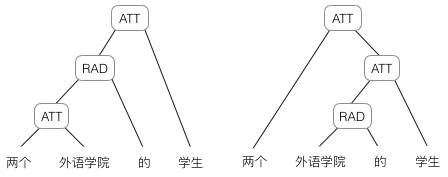上图显示了这句话的两个不同的语法解析树。可以看出来这句话有歧义，不同的语法解析树则对应了不同的意思。一个是『两个外语学院的/学生』，也就是学生可能有许多，但他们来自于两所外语学校；另一个是『两个/外语学院的学生』，也就是只有两个学生，他们是外语学院的。为了能够让模型区分出两个不同的意思，我们的模型必须能够按照树结构去处理信息，而不是序列，这就是递归神经网络的作用。当面对按照树/图结构处理信息更有效的任务时，递归神经网络通常都会获得不错的结果。

递归神经网络的一般结构为树状的层次结构，如图(a)所示，当递归神经网络的结构退化为线性序列结构（图b）时，递归神经网络就 等价于简单循环网络。分别对应上面的例子的两个结构。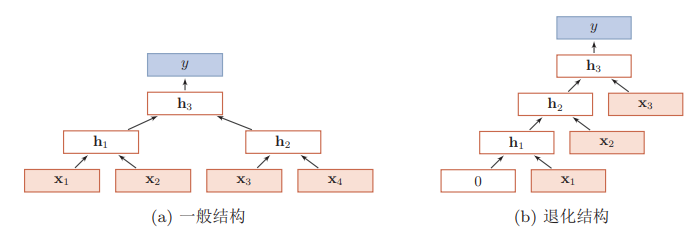7 LSTM

长短期记忆（Long Short-Term Memory，LSTM）网络 [Gers et al., 2000, Hochreiter and Schmidhuber, 1997]是循环神经网络的一个变体。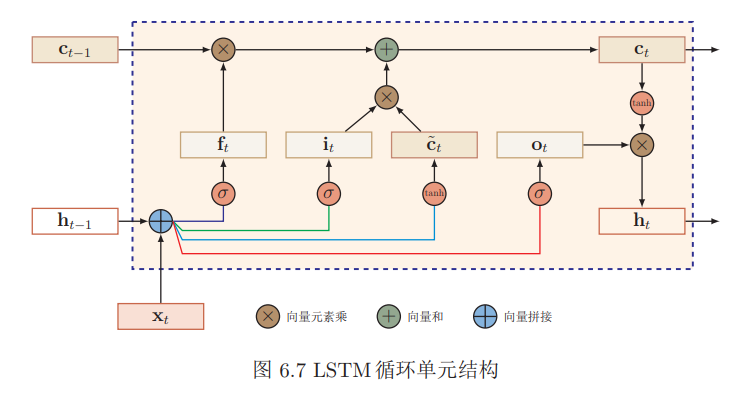LSTM网络主要改进在以下两个方面:

• 新的内部状态：LSTM 网络引入一个新的内部状态（internal state）专门进行 线性的循环信息传递，同时（非线性）输出信息给隐藏层的外部状态
• 门控机制：LSTM网络引入门控机制（Gating Mechanism）来控制信息传递的路径。

下面是它的详细说明：

长短时记忆网络的思路比较简单。原始RNN的隐藏层只有一个状态，即h，它对于短期的输入非常敏感。那么，假如我们再增加一个状态，即c，让它来保存长期的状态，那么问题不就解决了么？如下图所示：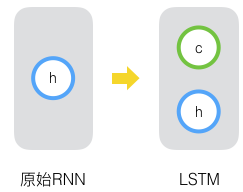新增加的状态c，称为单元状态(cell state)。我们把上图按照时间维度展开：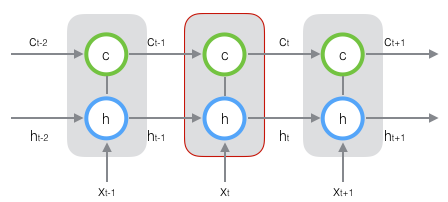上图仅仅是一个示意图，我们可以看出，在t时刻，LSTM的输入有三个：当前时刻网络的输入值、上一时刻LSTM的输出值、以及上一时刻的单元状态；LSTM的输出有两个：当前时刻LSTM输出值、和当前时刻的单元状态。注意都是向量

LSTM的关键，就是怎样控制长期状态c。在这里，LSTM的思路是使用三个控制开关。

• 第一个开关，负责控制继续保存长期状态c；
• 第二个开关，负责控制把即时状态输入到长期状态c；
• 第三个开关，负责控制是否把长期状态c作为当前的LSTM的输出。

分别对应以下三个门：

• 输入门 ：控制当前时刻的候选状态 有多少信息需要保存;
• 遗忘门 ：控制上一个时刻的内部状态 需要遗忘多少信息;
• 输出门：控制当前时刻的内部状态ct有多少信息需要输出给外部状态

三个开关的作用如下图所示：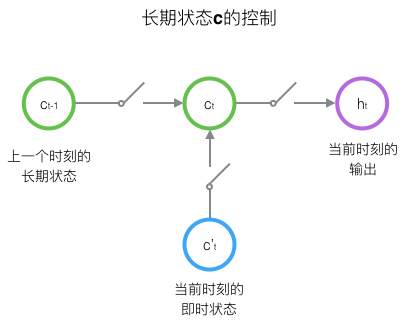8 门控循环单元网络

门控循环单元（Gated Recurrent Unit，GRU）网络 [Cho et al., 2014, Chung et al., 2014]是一种比LSTM网络更加简单的循环神经网络。

GRU对LSTM做了两个大改动：

• 将输入门、遗忘门、输出门变为两个门：更新门（Update Gate）和重置门（Reset Gate）。
• 将单元状态与输出合并为一个状态。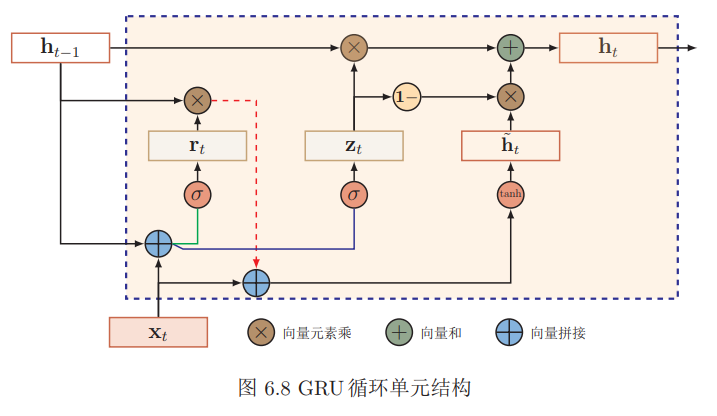Text-RNN的原理

假设训练集中所有文本/序列的长度统一为n，我们需要对文本进行分词，并使用词嵌入得到每个词固定维度的向量表示。对于每一个输入文本/序列，我们可以在RNN的每一个时间步长上输入文本中一个单词的向量表示，计算当前时间步长上的隐藏状态，然后用于当前时间步骤的输出以及传递给下一个时间步长并和下一个单词的词向量一起作为RNN单元输入，然后再计算下一个时间步长上RNN的隐藏状态，以此重复...直到处理完输入文本中的每一个单词，由于输入文本的长度为n，所以要经历n个时间步长。

基于RNN的文本分类模型非常灵活，有多种多样的结构。下面是两种典型的结构。

• structure v1: embedding--->BiLSTM--->concat final dropout/average all output--->softmax layer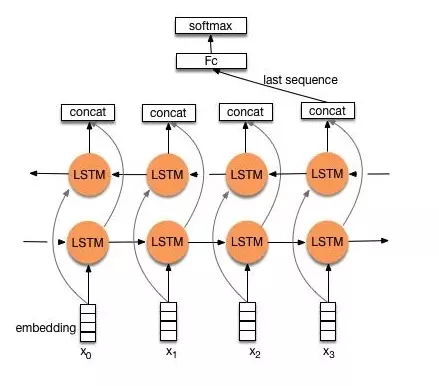一般取前向/反向LSTM在最后一个时间步长上隐藏状态，然后进行拼接，在经过一个softmax层(输出层使用softmax激活函数)进行一个多分类；或者取前向/反向LSTM在每一个时间步长上的隐藏状态，对每一个时间步长上的两个隐藏状态进行拼接，然后对所有时间步长上拼接后的隐藏状态取均值，再经过一个softmax层(输出层使用softmax激活函数)进行一个多分类(2分类的话使用sigmoid激活函数)。

• structure v2: embedding--->BiLSTM--->(dropout)--->concat dropout--->UniLSTM--->(dropout)-->softmax layer转存失败与之前结构不同的是，在双向LSTM(上图不太准确，底层应该是一个双向LSTM)的基础上又堆叠了一个单向的LSTM。把双向LSTM在每一个时间步长上的两个隐藏状态进行拼接，作为上层单向LSTM每一个时间步长上的一个输入，最后取上层单向LSTM最后一个时间步长上的隐藏状态，再经过一个softmax层(输出层使用softamx激活函数，2分类的话则使用sigmoid)进行一个多分类。

构建模型代码：

import tensorflow as tf
from tensorflow import keras

import numpy as np

imdb = keras.datasets.imdb

(train_data, train_labels), (test_data, test_labels) = imdb.load_data(num_words=10000)

print(sum(list(map(lambda x:len(x),train_data)))/len(train_data))
print(sum(list(map(lambda x:len(x),test_data)))/len(test_data))

max_len = 240
maxlen=max_len)

maxlen=max_len)

vocab_size = 10000

model = keras.Sequential()

model.summary()

loss='binary_crossentropy',
metrics=['acc'])

x_val = train_data[:10000]
partial_x_train = train_data[10000:]

y_val = train_labels[:10000]
partial_y_train = train_labels[10000:]

history = model.fit(partial_x_train,
partial_y_train,
epochs=40,
batch_size=512,
validation_data=(x_val, y_val),
verbose=1)

results = model.evaluate(test_data, test_labels)

print(results)

10 RCNN原理

TextRNN可以获取上下文信息，但是单向RNN是有偏的模型(biased model)，后面的词占得重要性更大。TextCNN是无偏的模型(unbiased model)，能够通过最大池化获得最重要的特征，但是特征提取器大小固定，设定小了容易造成信息丢失，设定大了造成巨大的参数空间。

为了解决TextRNN和TextCNN的模型缺陷，循环卷积神经网络(Recurrent Convolutional Neural Networks，RCNN)提出了：

• 双向循环结构：比传统的基于窗口的神经网络噪声要小，能够最大化地提取上下文信息；
• max-pooling池化层：自动决策哪个特征更有重要作用。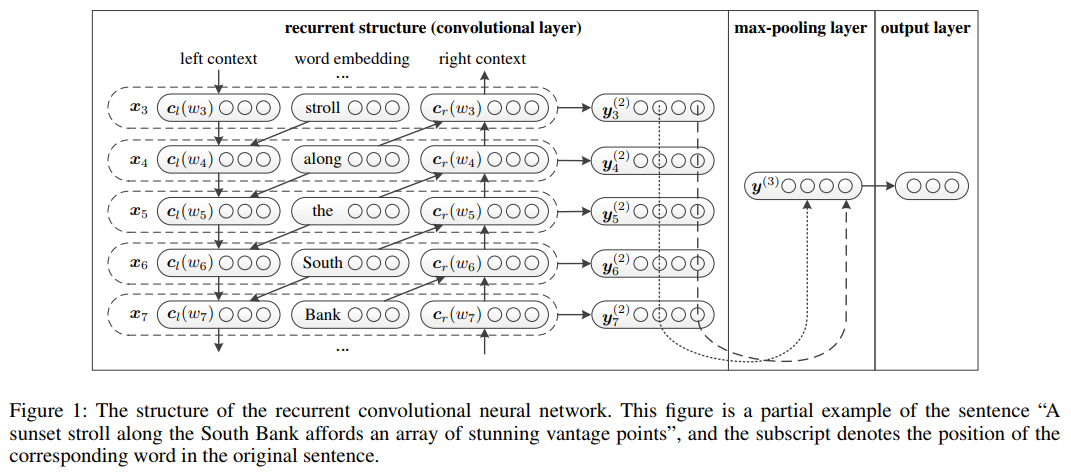推荐文章：

《神经网络与深度学习》

零基础入门深度学习

基于TextRNN的文本分类原理

Recurrent Convolutional Neural Networks for Text Classification

一文读懂文本分类基线深度模型从fastText、TextCNN、TextRNN、TextRCNN、HAN到Transformer

展开全文• 深度学习小白专场之循环神经网络和递归神经网络 全连接神经网络和卷积神经⽹网络，都只能单独的去处理单个的输入，且前后的输入之间毫无关系。但是在一些任务中，我们需要更好的去处理序列的信息，即前后的输⼊之间...

深度学习小白专场之循环神经网络和递归神经网络

全连接神经网络和卷积神经⽹网络，都只能单独的去处理单个的输入，且前后的输入之间毫无关系。但是在一些任务中，我们需要更好的去处理序列的信息，即前后的输⼊之间存在关系。比如，在理解一整句话的过程中，孤立理解组成这句话的词是不够的，我们需要整体的处理由这些词连接起来的整个序列；当我们处理视频时，我们也不能单独地仅仅分析每一帧，⽽要分析这些帧连接起来的整个序列。这就引出了了深度学习领域中另⼀类非常重要的神经网络：循环神经⽹网络（Recurrent Neural Network）。

1.循环神经网络

我们首先来看一下一个简单的循环神经⽹络图，它由输⼊层、一个隐藏层和⼀个输出层组成。我们可以看到，循环神经网络的隐藏层的值s不仅仅取决于当前这次的输入x，还取决于上一次隐藏层的值s，权重矩阵W就是隐藏层上一次的值作为这一次的输入的权重。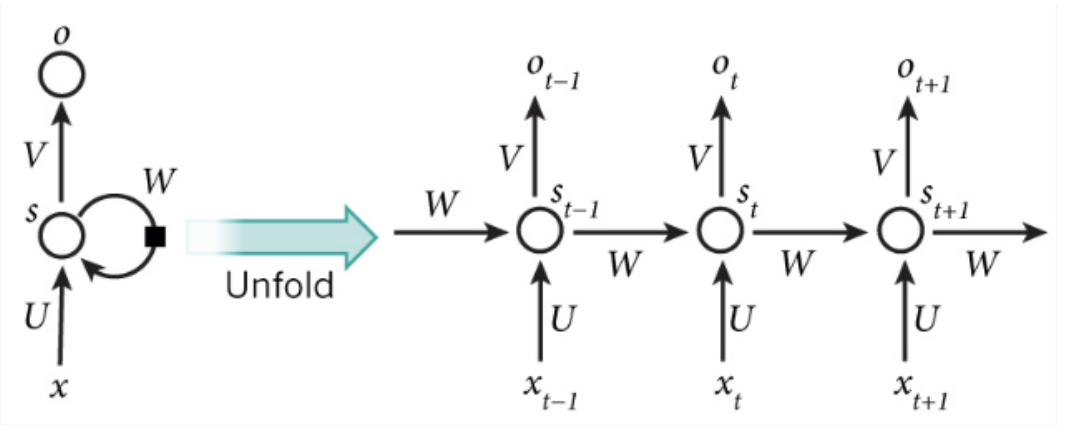从上⾯可以看出，循环神经⽹网络的输出值ot，是受前⾯历次输⼊入值xt、xt−1、xt−2. . . 的影响的， 这就是为什么循环神经网络可以往前看任意多个输入值的原因。
对比与卷积神经网络CNN一般用于处理网格化数据如一个图像的神经网络，循环神经网络RNN是一类用于处理序列数据的神经网络。

• 循环神经网络的训练：
循环神经网络训练的算法和卷积神经网络，前馈神经网络的训练算法-BP算法的原理是一样的，也包括3个步骤：
（1）前向计算每一个神经元的输出值
（2）反向计算每一个神经元的误差项，即误差对加权输入（加法器）的偏导数。这里的加法器就是wx+b
（3）计算每一个权重的梯度
注： 应用于展开图且代价为O（tao）的反向传播算法称为通过时间反向传播（BPTT）。
• CNN和RNN参数共享的区别：卷积的输出是一个序列，其中输出的每一项是相邻几项输入的函数，共享的概念体现在每个时间步中使用的相同的卷积核；而RNN是输出的每一项是前一项的函数。输出的每一项是对先前输出应用相同的更新规则而产生。
• RNN的几种设计模式：
（1）每个时间步都有输出，并且隐藏单元和隐藏单元之间有循环连接的循环网络
（2）每个时间步都有输出，只有当前时刻的输出到下一时刻的隐藏单元之间有循环连接的网络
（3）隐藏单元之间存在循环连接，但是读取整个序列后产生单个输出的循环网络

隐藏单元之间存在循环的网络非常强大但是训练代价也非常大。

• 由输出反馈到模型而后产生循环连接的模型可使用导师驱动过程进行训练。训练模型时，导师驱动过程不再使用最大似然准则。 导师驱动过程是一种训练技术，适用于输出与下一时间步的隐藏状态存在连接的RNN。

我们通常希望将RNN的输出解释为一个概率分布，并且通常使用与分布相关联的交叉熵来定义损失。例如均方误差是与单位高斯分布的输出相关联的交叉熵损失，例如前馈网络中所使用的。

• 循环网络为减少的参数数目付出的代价是优化参数可能变得困难。
• 我们可以将t=1，2，3···的向量x^(t)序列作为输入的RNN。另一种选择我们可以使用单个向量x作为输入。当x是一个固定大小的向量时，我们可以简单的将其看做产生y序列RNN的额外输入。
将额外的输入提供到RNN的一些常见方法是：
（1）在每个时刻作为一个额外输入
（2）作为初始状态h^(0)
(3)结合两种方式

将固定长度的向量x映射到Y序列上分布的RNN。这类RNN适应于很多任务，如图注，可以将单个对象作为模型的输入，然后产生描述图像的词序列。

2.双向RNN

对于语⾔模型来说，很多时候光看前面的词是不够的，我们需要看到一个词前后的词，这时候就要用到双向RNN了。下图为双向RNN示例：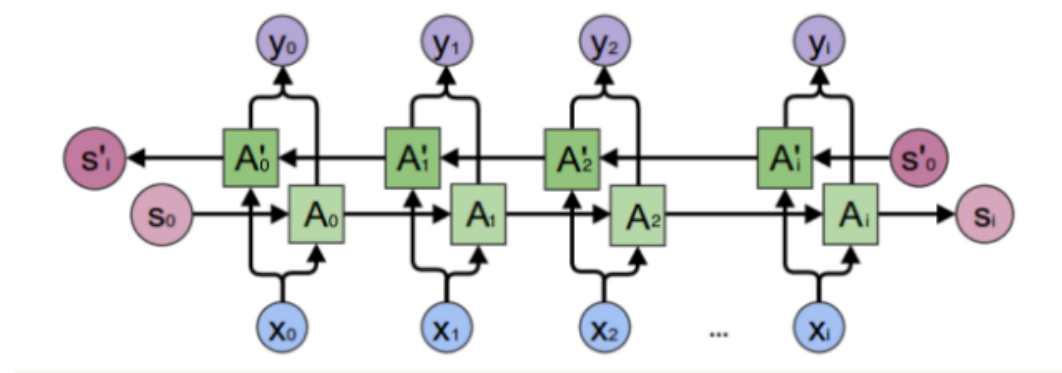我们可以看出一般的规律：正向计算时，隐藏层的值st与st−1有关；反向计算时，隐藏层的值s ′t与s ′ t+1有关；最终的输出取决于正向和反向计算的加和。

• 双向RNN允许输出单元同时依赖过去和未来且对时刻t的输入值最敏感的表示，而不必指定t周围固定大小的窗口。这是前馈网络、卷积网络或具有固定带下的线性缓存器的常规RNN所必须要做的。
• 在双向RNN中，循环h在时间上向前（向右）传播信息，循环g在时间上向后（向左）传播信息。
但是实践中，上面介绍的这两种RNN并不能处理较长的序列，主要是由于梯度消失和梯度爆炸的问题。使用其他结构的RNNs可以避免梯度消失问题，⽐如长短时记忆网络（LTSM）和Gated Recurrenr Unit（GRU），这是最流行的做法。同时避免使用饱和性激活函数比如sigmoid和tanh函数而采用ReLu函数也可以避免梯度消失问题。对于梯度爆炸问题我们可以通过设置梯度阈值来避免。

3.基于编码-解码的序列到序列架构

• 我们还可以训练RNN使得输入序列可以变为不一定等长的输出序列。在这很多场景中都有应用，如语音识别、机器识别、或者问答，其中训练集的输入和输出序列的长度通常不相同。我们通常称为将这样的输入称为“上下文”。

4.递归神经网络

• 递归神经网络代表循环网络的另一个扩展，它被构造为深的树状结构而不是RNN的链状结构。因此是不同的计算图。
• 递归神经网络的一个明显优势是，对于具有相同长度tao的序列，深度可以急剧地从tao减小到log（tao），这可能有助于解决长期依赖。
展开全文深度学习 循环神经网络 递归神经网络
• 1.循环神经网络(recurrent neural...2.递归神经网络(recursive neural network)递归神经网络是空间上的展开,处理的是树状结构的信息,是无环图,模型结构如下: recursive: 空间维度的展开,是一个树结构,比如nlp里某. ...

1.循环神经网络(recurrent neural network)是时间上的展开,处理的是序列结构的信息,是有环图,模型结构如下: recurrent: 时间维度的展开,代表信息在时间维度从前往后的的传递和积累…
2.递归神经网络(recursive neural network)递归神经网络是空间上的展开,处理的是树状结构的信息,是无环图,模型结构如下: recursive: 空间维度的展开,是一个树结构.

展开全文神经网络 深度学习
• 最简单的循环递归神经网络，适合刚接触神经网络的初学者学习。
• 即使不是 NLPer，现实中依然会面对很多序列...全文内容来自 Ian Goodfellow, Yoshua Bengio Aaron Courville 3位大老爷的作品“Deep Learning”的其中一章“Sequence Modeling: Recurrent and Recursive Nets”。

即使不是 NLPer，现实中依然会面对很多序列问题。

全文内容来自 Ian Goodfellow, Yoshua Bengio 和 Aaron Courville 3位大老爷的作品“Deep Learning”的其中1章“Sequence Modeling: Recurrent and Recursive Nets” 1

1986年 Rumelhart 等人提出循环神经网络。循环网络与多层网络相比，会共享每层的权重，从而能够扩展和应用网络于不同长度的序列案例，以及泛化这些案例。当1片特定的信息可以在序列中多处出现时，这样的共享尤为重要。比如，有两句话：“I went to Nepal in 2009”和“In 2009, I went to Nepal.”如果要求模型提取叙述者哪一年去的 Nepal，不管是输入句子的第2处还是第6处，2009 都是应识别的信息片。传统全连接前向网络对每处输入的特征都有单独的参数，所以要单独学习输入句子每一处的所有语法。相比之下，循环网络在不同时间步间共享权重

现在很多“神经网络”的字眼已被“网络”替换。且后面不会涉及神经元的内容，所以一概用“网络”。

相关的想法是1维时间序列间用卷积。卷积方法是延时神经网络的基础 (1988~1990)。卷积操作允许网络在时间轴上共享参数，但为浅层网络 (输出序列的每个节点输出为很多相邻节点输入的函数)。每个时间步上用相同的卷积核。

循环网络以不同的方式共享参数。节点输出为前面节点输出的函数，节点的输出由前面节点输出用相同的更新规则产生。通过很深的计算图来共享权重，从而形成循环。

为简化描述，RNN 看作对1个包含时间步索引 t $t$ (从1$1$ τ $\tau$) 的向量 x(t) $x^{(t)}$的序列的操作。实际上，循环网络通常操作小的序列块 (batch)。这里简化表示，剔除块索引。此外，时间步长的索引仅指序列中的位置，而不一定指现实世界中的时间通道。 RNN 也可以应用于2维空间数据 (如图像) 。甚至当数据涉及时间时，如果提供观察到的整个序列给网络，那么网络可能要有及时回顾过去的连接。

循环代表某变量的过去值对未来值的影响。用循环的计算图定义循环网络。

1. 展开计算图 (Computational Graph)

计算图是形成一系列计算结构 (如涉及映射输入和参数到输出和损失) 的1种方式。把1个循环/递归计算展开为1个有重复结构 (对应1连串事件) 的图，从而实现深度网络结构间的参数共享。

例如，考虑动态系统的经典形式：

s(t)=f(s(t1);θ)

其中， s(t) $s^{(t)}$为系统的状态。

上述系统是循环的，因为时刻 t $t$的状态s$s$的定义回溯到时刻 t1 $t-1$的状态 s $s$的定义。

对于有限时间步τ$\tau$，用定义 τ1 $\tau - 1$次来展开成图。当 τ=3 $\tau=3$ (时间步) 时，有

s(3)=f(s(2);θ)=f(f(s(1);θ);θ)

展开后的表达式可以表示为传统的 有向非循环计算图再举1例，外部输入 x(t) $x^{(t)}$驱动的动态系统：

s(t)=f(s(t1),x(t);θ)

现在的状态 s $s$包含所有过去序列的信息。

循环网络可用很多不同方式搭建。几乎任何函数都可看作1个前向网络，本质上任何涉及循环的函数都能当作1个循环网络 (RNN)

为表明状态即为网络的隐藏单元，上述方程重写为：

h(t)=f(h(t1),x(t);θ)典型的 RNN 增加从状态 h $h$读取信息的输出层来预测。

当训练循环网络来用过去预测未来时，网络通常学习去用状态h(t)$h^{(t)}$作为与截止到 t $t$的过去输入序列的任务相关方面的有损总结 (lossy summary)，因为该总结映射任意长度的序列(x(t),x(t1),x(t2),...,x(2),x(1))$(x^{(t)},x^{(t-1)},x^{(t-2)},...,x^{(2)},x^{(1)})$到固定长度的向量 h(t) $h^{(t)}$。取决于训练要求，该总结可能有选择地更明确地保持过去序列的某些部分。比如，RNN 用于统计语言建模时，通常给定前面的句子去预测下一个单词，它可能没必要保存截至时间 t $t$的输入序列的所有信息，只保存足够预测剩下语句的信息即可。

画 RNN 有2种方式。

• 每部分仅包含1个节点。网络定义为1个实时操作的电路，该电路物理部分的当前状态会影响未来状态 (如上左图示)。黑色方块表示状态从时间t$t$到时间 t+1 $t+1$发生了1个时间步长的延时。

• 展开的计算图。每部分由许多不同的变量表示，每个时间步长1个变量 (该变量表示某时刻该部分的状态，计算图中画成单独的节点)。展开之意为映射左图中的电路至右图带重复部分的计算图。展开图的长度取决于序列长度。
• 用函数 g(t) $g^{(t)}$表示 t $t$时间步后的展开循环：

h(t)=g(t)(x(t),x(t1),x(t2),...,x(2),x(1))=f(h(t1),x(t);θ)

函数 g(t) $g^{(t)}$的输入为整个过去的序列 (x(t),x(t1),x(t2),...,x(2),x(1)) $(x^{(t)},x^{(t-1)},x^{(t-2)},...,x^{(2)},x^{(1)})$，输出为当前状态，但展开的循环结构分解 g(t) $g^{(t)}$为函数 f $f$的重复应用。展开有两种好处：

• 不管序列长度，学习到的模型的输入大小相同，因为它被指定从1个状态转到另1个状态，而不是可变长度状态的历史。
• 每个时间步，参数相同，转换函数f$f$相同。

以上好处使得单个模型 f $f$能在所有时间步和所有序列长度上操作。学习单个模型可以泛化到训练集中未出现的序列长度，参数共享使模型估计用到更少的训练样本。

循环图和展开图各有用途。循环图简明；展开图清晰地描述计算过程，并有助于说明随时间前向 (计算输出和损失) 和反向 (计算梯度) 的信息流。

2. 循环网络 (Recurrent Neural Networks)

循环网络的重要设计模式包括：

• 1个时间步产生1个输出，隐含单元之间循环连接 (下图左)。
• 1个时间步的输出连入另1时间步的隐含单元 (下图中)。
• 隐含单元之间循环连接，读取整个序列，最后1个隐含单元产生1个输出 (下图右)。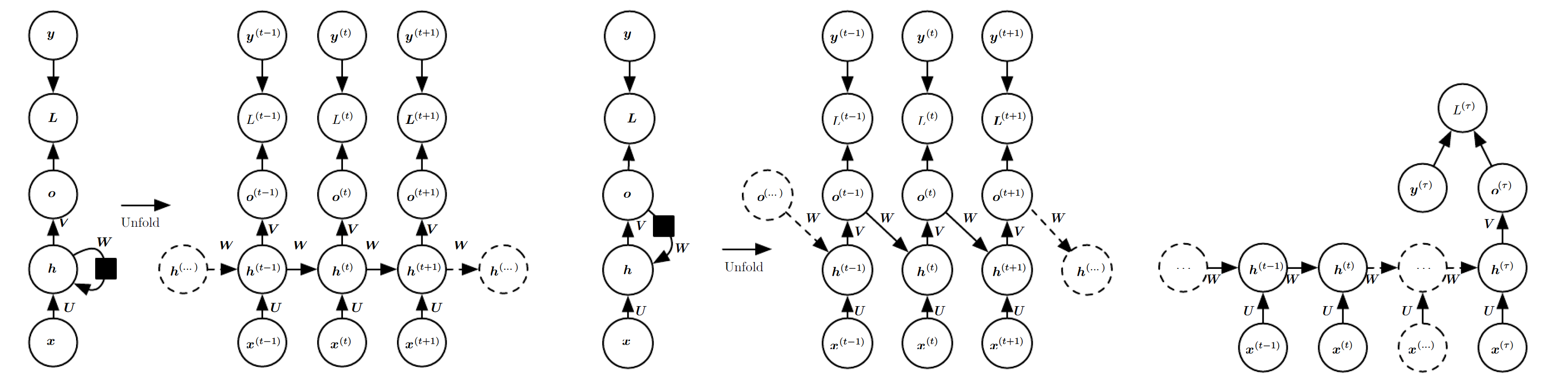上图左对应的 RNN 是通用的。理论上的 RNN 被证用 RNN 的激活函数和权重 (无限精度的有理数) 可模拟1个无限长的堆栈。

假定隐含单元选用双曲正切激活函数，输出为离散值，如预测单词或字符。离散变量的每个可能值的未归一化对数概率表示离散变量的输出o$o$，输入给 softmax 回归，输出归一化的概率向量 y^ $\hat y$。前向传播的起始状态指定为 h(0) $h^{(0)}$。从时间步 t=1 $t=1$~ τ $\tau$，用下面的更新方程：

a(t)=b+Wh(t+1)+Ux(t)h(t)=tanh(a(t))o(t)=c+Vh(t)y^(t)=softmax(o(t))

其中， b $b$c$c$为偏置， U $U$V$V$ W $W$为输入到隐含单元，隐含单元到输出，隐含单元到隐含单元的连接权重。这里，输入序列与输出序列的长度相同。全部损失为所有时间步的损失之和。比如，如果给定x(1),...,x(τ)$x^{(1)},...,x^{(\tau)}$ L(t) $L^{(t)}$ y(t) $y^{(t)}$的负对数似然损失，则
L({x(1),...,x(τ)},{y(1),...,y(τ)})=t=1τL(t)=t=1τlogpmodel(y(t)|{x(1),...,x(τ)})

其中， pmodel(y(t)|{x(1),...,x(τ)}) $p_{model}\left(y^{(t)}|\{x^{(1)},...,x^{(\tau)}\}\right)$为模型的输出向量 y^(t) $\hat y^{(t)}$预测为 y(t) $y^{(t)}$的概率。该损失函数的梯度计算费时。见上图左，梯度计算涉及展开图上的从左往右的前向传播，紧随从右往左的反向传播。运行时间为 O(τ) $O(\tau)$，因前向传播图本质上为序列，所以不能并行减少时间。先计算前1个时间步才能得到后面的每个时间步。前向计算的状态会被保存直到反向计算时重用，所以内存开销也为 O(τ) $O(\tau)$应用于代价为 O(τ) $O(\tau)$的展开图的反向传播算法被称为穿过时间的反向传播 (BPTT)。隐含单元间的循环网络因此很强大但训练困难。

(1) 老师强迫 (Teacher Forcing) 与 输出循环的网络

某1时间步的输出仅循环连接到下1时间步的隐含单元的网络是严格弱的，因为它缺少隐含单元到隐含单元的循环连接。比如，它不能模拟1个通用的图灵机。因为它缺少隐含层到隐含层间的循环，所以网络用于预测未来时，输出单元要求捕捉过去所有的信息。显式训练输出单元来匹配训练集合的目标不太可能捕获关于输入过去历史的必要信息，除非用户了解系统的全部状态且把它作为训练目标的一部分。

对时间步 t $t$的预测与其训练目标比较的任何损失函数来说，消除隐含单元到隐含单元的循环可解耦所有的时间步。单独计算每个时间步t$t$的梯度可并行训练，不必先算前面时间步的输出，因为训练集已提供输出的完美值。

从输出到状态的循环连接模型可能用“老师强迫”训练。训练时，模型接受真实输出 y(t) $y^{(t)}$为时间步 t+1 $t+1$的输入，老师强迫是遵从最大似然标准的过程。检查两个时间步的序列，条件最大似然标准为：

logp(y(1),y(2)|x(1),x(2))=logp(y(2)|y(1),x(1),x(2))+logp(y(1)|x(1),x(2))

本例中，时间步 t=2 $t=2$时，给定训练数据中的截至 t $t$x$x$序列和 y $y$值，训练模型来最大化y(2)$y^{(2)}$的条件概率。训练时指定最大似然，而非输出自循环。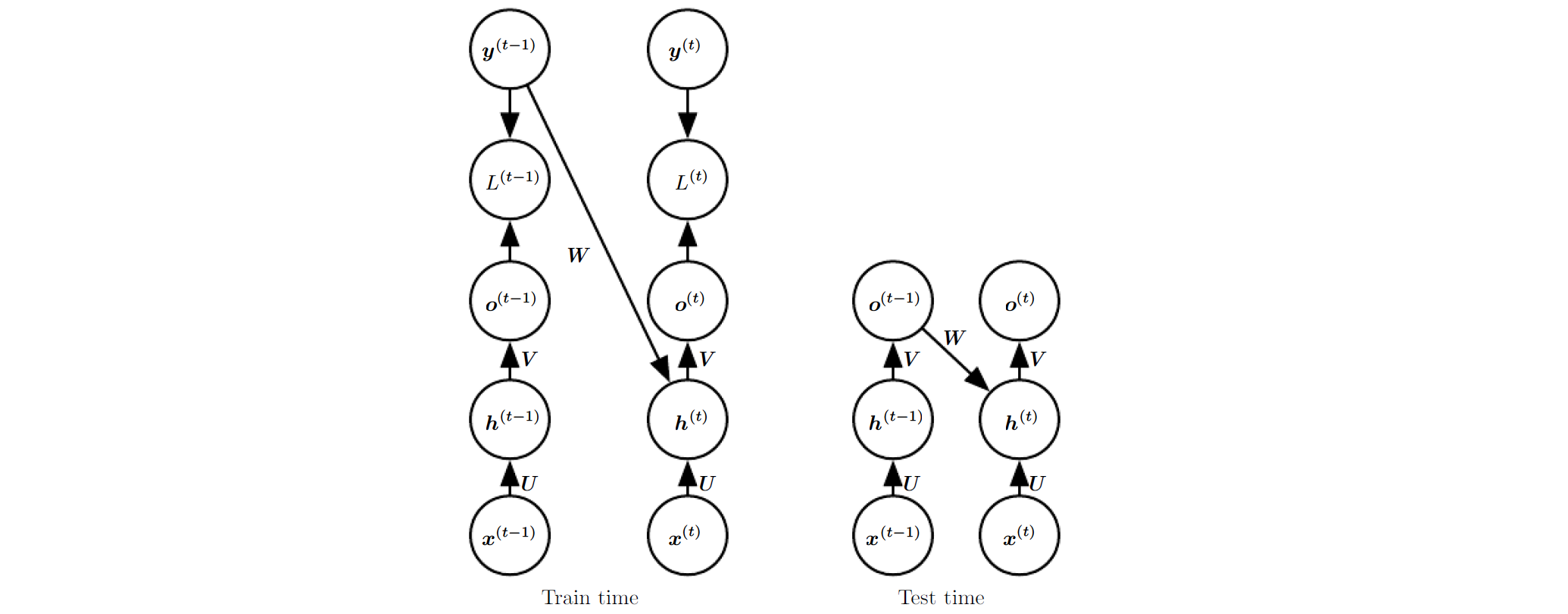隐含单元间缺少连接的模型上用老师强迫可避免穿过时间的反向传播。老师强迫也能用于输出与状态循环连接的模型。然而，一旦隐含单元变成1个前面时间步的函数，BPTT算法是必要的。一些模型同时用老师强迫与 BPTT。

如果网络用于开环模式 (网络输出反馈给输入)，严格老师强迫会出问题。训练时网络输入与测试时输入可以有很大差异。

训练时，同时用老师强迫的输入与未来几个时间步后预测的正确目标作输入。另外1种缓和训练与测试输入间的差异，随机选择泛化输出或实际数据作输入。该方式用课程学习策略，逐渐用更多的泛化值作输入。

“老师强迫”原意可这样理解：假设状态为学生的学习态度，输入为学生的作业，输出为尽可能好的期末考试成绩。老师没办法让学渣突然爱上学习，所以，一般会通过强迫学生写作业这样的外在手段来提高学生的成绩。

(2) 循环网络中的梯度计算

循环网络的梯度计算比较直接。展开的计算图上用泛化的反向传播算法即可。展开图上的反向传播应用被称为穿过时间的反向传播 (BPTT) 算法。反向传播得到的梯度接着可用作任何通用的基于梯度的技术来训练 RNN。

BPTT 为 RNN 方程 (上述 RNN 的正向传播) 计算梯度。以重要设计模式中的左图为例 (隐含层单元间循环连接)，计算图的节点包括 U,V,W,b,c,x(t),h(t),o(t),L(t) $U,V,W,b,c,x^{(t)},h^{(t)},o^{(t)},L^{(t)}$

a. 沿时间步的正向梯度

递归地计算每个节点 N $N$的梯度损失NL$\nabla_{N}L$。首先：

LL(t)=1

并不是 L=L(t) $L=L^{(t)}$ L $L$为所有时间步的L(t)$L^{(t)}$的和。

假定 softmax 回归输入 o(t) $o^{(t)}$输出 y^ $\hat y$，且损失是真实目标 y(t) $y^{(t)}$的负对数似然。时间步 t $t$上输出的梯度o(t)L$\nabla_{o^{(t)}}L$，对所有的 i,t $i,t$

(o(t)L)i=LL(t)L(t)y^(t)iy^(t)io(t)i=1×1y^(t)i×y^(t)i×(1y^(t)i)=y^(t)i1

其中， i $i$为输出向量o(t)$o^{(t)}$的第 i $i$个元素。

b. 沿时间步的反向梯度

从序列末端开始，时间步τ$\tau$ h(τ) $h^{(\tau)}$仅派生了 o(τ) $o^{(\tau)}$ (不存在 h(τ+1) $h^{(\tau+1)}$)，有：

h(τ)L=VToτL

沿时间轴往回迭代 (从 τ1 $\tau-1$ 1 $1$) 来反向传播梯度。注意到h(t)$h^{(t)}$派生了 o(t) $o^{(t)}$ h(t+1) $h^{(t+1)}$。所以梯度为：
h(t)L=(h(t+1)h(t))T(h(t+1)L)+(o(t)h(t))T(o(t)L)=WT(h(t+1)L)diag(1(h(t+1))2)+VT(o(t)L)

其中， diag(1(h(t+1))2) $diag\left(1-\left(h^{(t+1)}\right)^2\right)$为包含元素 1(h(t+1)i)2 $1-(h_i^{(t+1)})^2$的对角矩阵。这是第 i $i$个隐含单元在时间步t+1$t+1$的双曲正切的 Jacobian 矩阵。

h(t+1)=tanh(b+Wh(t)+Ux(t+1)) $h^{(t+1)}=tanh(b+Wh^{(t)}+Ux^{(t+1)})$

c. 单个时间步内的参数梯度

获得计算图的内部节点的梯度后，可获得参数节点上的梯度。因参数在许多时间步间共享，所以当微积分操作涉及这些参数时必须小心处理。用反向传播方法得到计算图中单个边 (edge) 的梯度。然而， Wf $\nabla_W f$考虑 W $W$对基于计算图中所有边的f$f$的分布。为方便解释，引入伪变量 W(t) $W^{(t)}$ (定义为 W $W$在时间步t$t$的复制)。用 W(t) $\nabla W^{(t)}$表示时间步 t $t$的权重分布的梯度。

{c,V}:o(t)=c+Vh(t)$\{c,V\}:o^{(t)}=c+Vh^{(t)}$
{b,W,U}:h(t)=tanh(b+Wh(t1)+Ux(t)) $\{b,W,U\}:h^{(t)}=tanh(b+Wh^{(t-1)}+Ux^{(t)})$

这样，内部参数的梯度为：

cL=t=1τ(o(t)c)To(t)L=t=1τo(t)LVL=t=1τiLo(t)iVo(t)i=t=1τ(o(t)L)h(t)TbL=t=1τ(h(t)b(t))Th(t)L=t=1τdiag(1(h(t))2)h(t)LWL=t=1τiLh(t)iW(t)h(t)i=t=1τ((h(t)L)Tdiag(1(h(t))2))Th(t1)TUL=t=1τiLh(t)iUh(t)i=t=1τ((h(t)L)Tdiag(1(h(t))2))Tx(t)T

(3) 循环网络视为有向图模型 (Directed Graphical Models)

a. 图模型参数化

上例的循环网络的损失 L(t) $L^{(t)}$为训练目标 y(t) $y^{(t)}$ o(t) $o^{(t)}$间的交叉熵。正如1个前向网络，原则上应使用1个循环网络中几乎所有的损失。应选择基于任务的损失。通常希望视 RNN 的输出为1个概率分布，且与该分布相关的交叉熵定义为损失。均方差是1个输出为正态分布的交叉熵。

训练 RNN 时，给定过去输入，最大对数似然来估计下1个序列元素 y(t) $y^{(t)}$的条件分布：

logp(y(t)|x(1),...,x(t))

若该模型包含从1个时间点到另1个时间点输出的连接，有：
logp(y(t)|x(1),...,x(t),y(1),...,y(t1))

当过去的 y $y$值不影响下1时间步的预测时，有向图模型不包含从过去的y(i)(i=1,...,t1)$y^{(i)}(i=1,...,t-1)$到当前 y(t) $y^{(t)}$的边；否则包含所有这样的边。直接参数化该图模型 (下图左) 效率低，输入和参数的数目增长快。RNN 具有相同的全连接，且参数化更高效。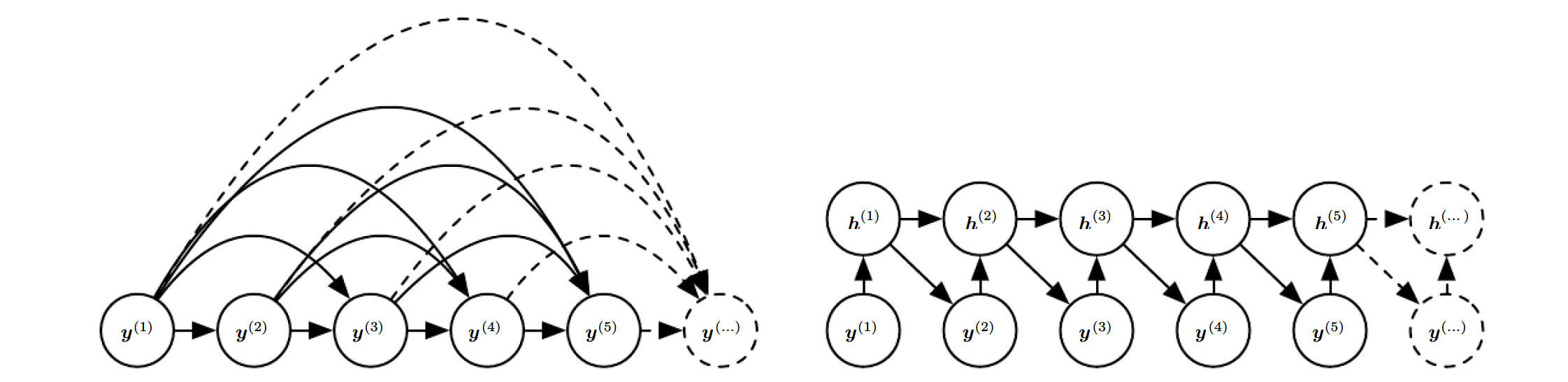考虑 RNN 为1个随机标量序列 Y={y(1),...,y(τ)} $Y=\{y^{(1)},...,y^{(\tau)}\}$，无输入 x $x$，时间步t$t$的输入仅为时间步 t1 $t-1$的输出 (上图右)。这样，RNN 模型定义为1个关于变量 y $y$的有向图模型。用条件概率的链式规则参数化y$y$的联合分布：

P(Y)=P(y(1),...,P(y(τ)))=P(y(τ)|y(τ1),...,y(1))P(y(τ1)|y(τ2),...,y(1))...P(y(2)|y(1))P(y(1))=t=1τP(y(t)|y(t1),...,y(1))

t=1 $t=1$时， P(Y)=P(y(1)) $P(Y)=P(y^{(1)})$。因此， {y(1),...,y(τ)} $\{y^{(1)*},...,y^{(\tau)*}\}$的负对数似然为：
L=t=1τL(t)=t=1τlogp(y(t)=y(t)|y(t1),...,y(1))

图模型的边表明变量与其它变量直接的依赖关系。删除节点间相互作用弱的边可使许多图模型的统计与计算更高效。如 Markov 假设 (图模型应仅包含 {y(tk),...,y(t1)} $\{y^{(t-k)},...,y^{(t-1)}\}$ y(t) $y^{(t)}$的边，而非包含所有过去的边)。然而，所有过去的输入应对序列的下一个元素有影响。当遥远过去的某个 y(i) $y^{(i)}$值对 y(t1) $y^{(t-1)}$无影响，但却对 y(t) $y^{(t)}$产生影响时，RNN 更有用。

b. 图模型解释 RNN

视 RNN 为1个具有完整的图结构，可表示任意 y $y$值对间的直接依赖的图模型。排除隐含单元h(t)$h^{(t)}$的 RNN 模型可解释为完整的图。

视隐含单元 h(t) $h^{(t)}$为随机变量得出 RNN 的概率图模型结构。概率图模型中包含隐含单元显示 RNN 极为有效地参数化观测值的联合分布。假定用表格表示任意1个离散量的联合分布 (每个元素给出事件发生的概率)，如果 y $y$可取k$k$个不同值，表格有 O(kτ) $O(k^\tau)$个参数。相比之下，参数共享使 RNN 有 O(1) $O(1)$个参数 (为序列长度的函数)。RNN 参数数目可能会被调整来控制模型容量，而不必按序列长度扩展。RNN 每个时间步上循环使用相同的 f $f$θ$\theta$图模型中插入隐含单元 h(t) $h^{(t)}$节点解耦了过去与未来，为它们之间的中间量。遥远过去的某个变量 y(i) $y^{(i)}$可能通过影响 h $h$来影响y(t)$y^{(t)}$

有效参数化后的图模型难以预测序列中间的丢失值。减少参数数目后循环网络却难以优化参数。

循环网络的权重共享的前提是假设相同的参数可用于不同的时间步。给定时间步 t $t$的变量，时间步t+1$t+1$的变量的条件概率分布是静态的，即前后时间步的关系与 t $t$无关。原则上，t$t$可用作每个时间步的额外输入，从而学习者能够在尽可能共享权重的同时发现任何的时变性。这已经强过每个时间步 t $t$对应1个条件概率分布。但出现新的时间步时，仍要推断网络新增时间步的时变性。

c. 序列长度

在对模型采样前，先确定序列长度：

• 改变模型输入
• 改变模型输出

当输出y$y$为单词中的字符时，加1个特殊标志来结束1个序列。特殊字符产生时，采样过程终止。训练集中，每个训练样本的 x(τ) $x^{(\tau)}$后面添加特殊字符输入

添加二值输出，决定每个时间步继续或终止产生序列。该方法更通用，不会局限于字符序列，还可用于实数序列等。新的输出单元为交叉熵损失训练出的 Sigmoid 单元，来最大化每个时间步上继续还是终止序列正确预测时的对数似然概率。

添加序列长度 τ $\tau$，用模型来预测 τ $\tau$。先采样 τ $\tau$，再采样 τ $\tau$长度的序列样本。该方法要求在每个时间步的循环更新处添加额外的输入，来提示是否接近1个序列的末端。该输入可以是序列长度 τ $\tau$或者 τt $\tau-t$ (剩余的时间步)，从而避免 RNN 产生序列结束前突然终止的可能。强行分解序列的联合概率分布：

P(x(1),...,P(x(τ)))=P(x(1),...,x(τ)|τ)P(τ)

(4) 取决于 RNN 上下文的训练模型

任何变量为 P(y;θ) $P(y;\theta)$的模型都可解释为条件分布为 P(y|ω) $P(y|\omega)$ ( ω=θ $\omega=\theta$)的模型。如果 ω $\omega$为输入 x $x$的函数，那么P(y|x)$P(y|x)$能表示与 P(y|ω) $P(y|\omega)$分布相同的模型。

I. 向量输入

前面讨论过 x(t) $x^{(t)}$ 序列 ( t=1,...,τ $t=1,...,\tau$) 为输入的 RNN。另1种方法，添加单个定长的向量 x $x$ (序列的元素) 为 RNN 的额外输入。为 RNN 提供额外输入的常用方法：

• 作为每个时间步的额外输入
• 作为初始状态h(0)$h^{(0)}$

• 两者结合
•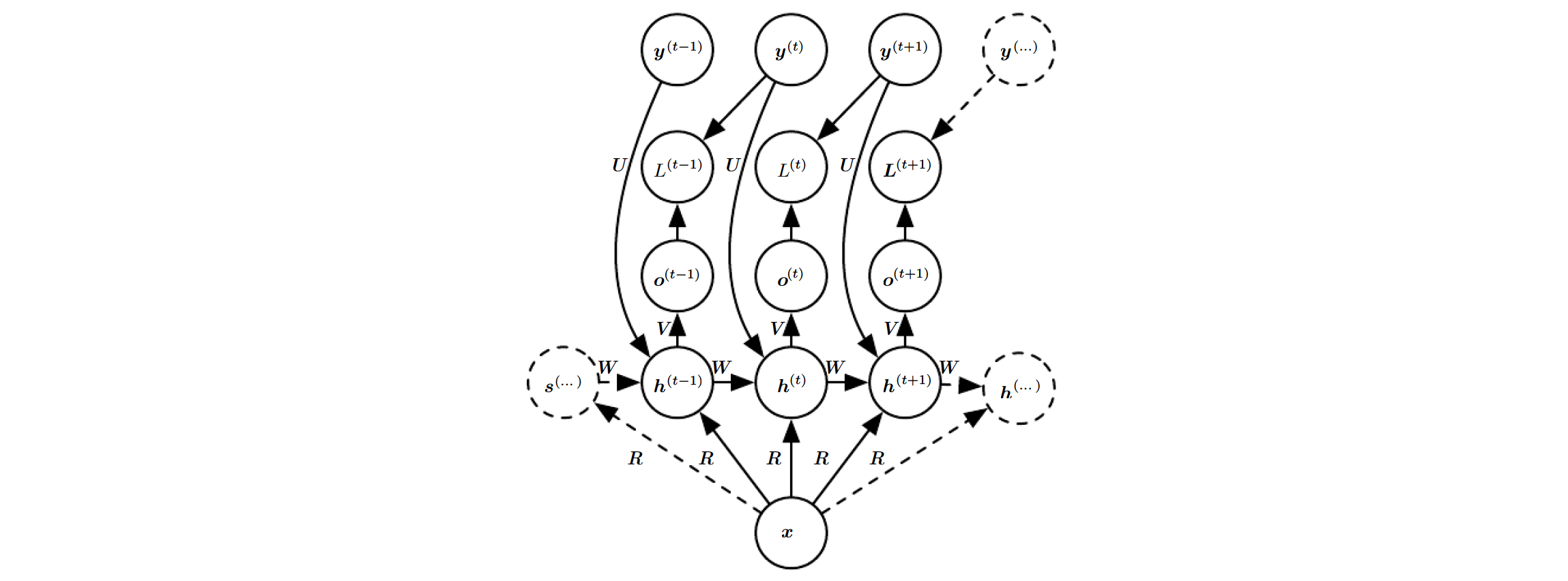引入权重矩阵 R $R$，每个时间步上隐含单元的输入为xTR$x^TR$ x $x$的选择决定了每个隐含单元新的偏置xTR$x^TR$是否有效。非条件模型 (无输入 x $x$x(t)$x^{(t)}$) 的参数 θ $\theta$变为 ω $\omega$后仍然保持不变，除了 ω $\omega$中的偏置参数现在变为输入 x $x$的函数。

前面讨论的输入为{x(1),...,x(τ)}$\{ x^{(1)},...,x^{(\tau)} \}$，现在讨论的输入为 {xTR,...,xTR} $\{ x^TR,...,x^TR \}$

该 RNN 适用于给图像加标题 (输入为单幅图像，输出为描述图像的语句)。观测序列的元素 y(t) $y^{(t)}$既为当前时间步的输入，也为训练时前面时间步的目标。

II. 向量序列输入

RNN 输入为向量序列 {x(1),...,x(τ)} $\{x^{(1)},...,x^{(\tau)}\}$时，假设输出 y(t) $y^{(t)}$条件独立,，则分解为 (见“图模型参数化”部分)：

P(y(1),...,y(τ)|x(1),...,x(τ))=t=1τP(y(t)|y(t1),...,y(1),x(1),...,x(τ))=t=1τP(y(t)|x(1),...,x(τ))

假设不条件独立，时间步 t $t$的输出连到时间步t+1$t+1$的隐含单元。此时，模型能表示任意序列 y $y$的概率分布。给定序列x$x$来描述另1个序列 y $y$分布的模型时，限制两个序列长度相同。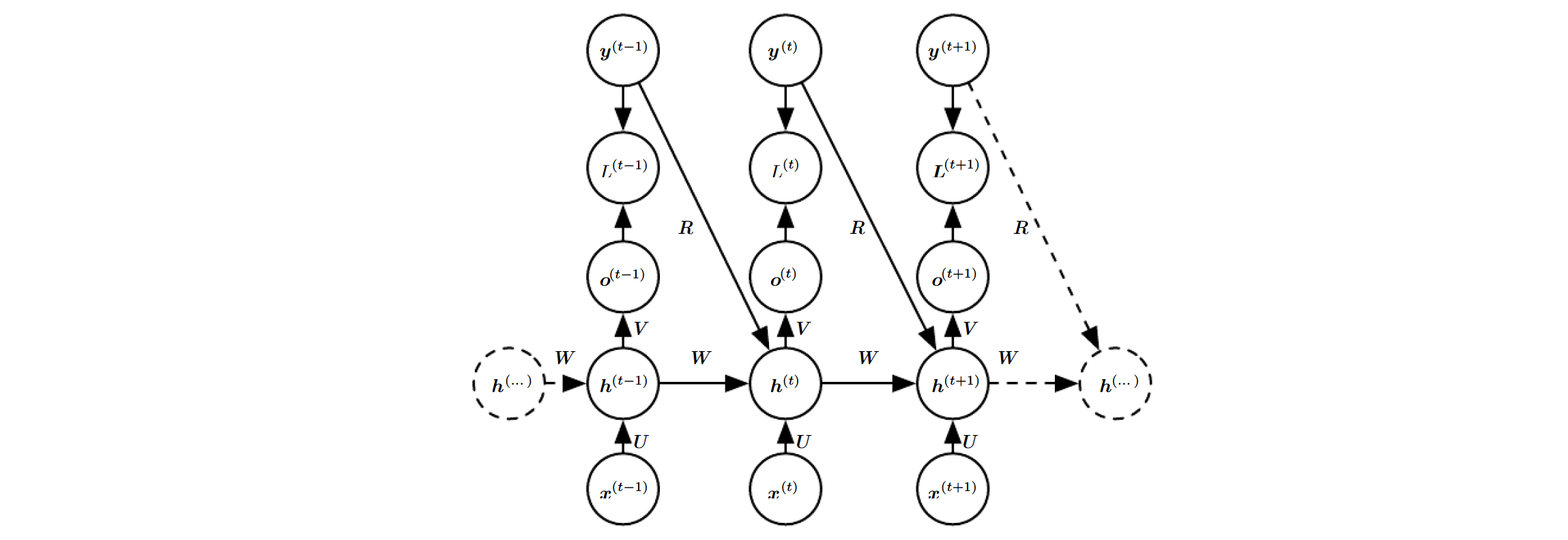3. 双向 RNN

大标题 (“1., 2., 3. …”) 为原作者给的，以 RNN 类型来区分；小标题 (“I., II., III. …”) 是我给的，以 实际问题的输入类型区分。所以，唯独这里的小标题的索引会不连贯。

III. 完整向量序列输入

许多应用的预测输出y(t)$y^{(t)}$可能取决于完整的输入序列。如语音识别中，当前音位的正确解释可能因协同发音取决于后几个音位，且甚至可能因单词间语境取决于后几个单词：须往过去或未来看得更远来区分当前单词的两个声学相似的解释。手写识别和其它序列到序列的学习类似。

1997 年 Schuster 和 Paliwal 发明双向 RNN。2005~2013 年间在手写体识别，语音识别和生物信息等方面的应用取得巨大成功。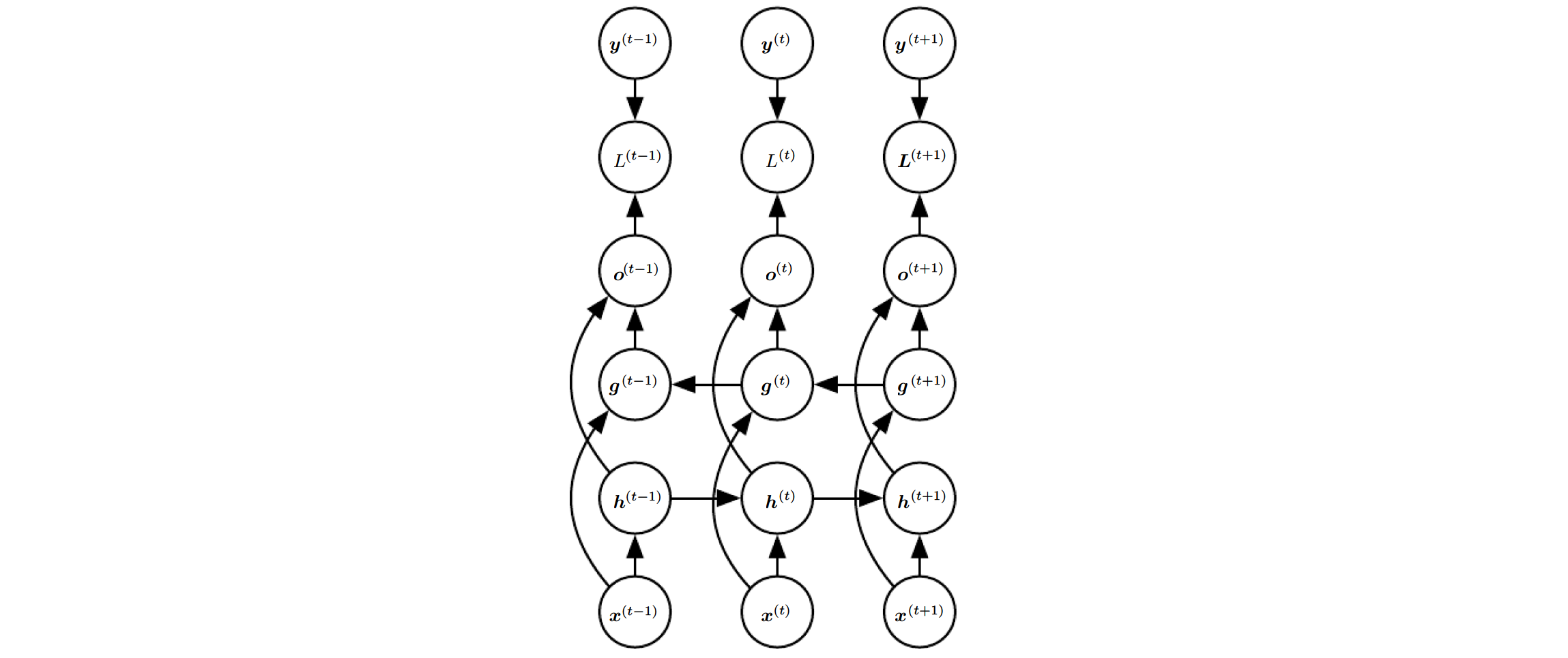双向 RNN 结合正向 RNN 和 反向 RNN。正向 RNN 从序列起点随时间移动至终点，反向 RNN 从序列终点随时间移动至起点。正反向 RNN 的状态分别为 h(t) $h^{(t)}$ g(t) $g^{(t)}$过去和未来都会影响输出单元 o(t) $o^{(t)}$，但输出对时间步 t $t$周围的输入最为敏感，且不必指定围绕时间步t$t$的固定大小的窗口。

用4个 RNN 可扩展至2维输入 (如图像)。每个 RNN 沿1个方向 (上，下，左和右) 移动。输入 2D 网格上的每个点 (i,j) $(i,j)$，当每个 RNN 的输入序列足够长时，输出 O(i,j) $O(i,j)$能捕获大部分局部信息。与卷积网络相比，RNN 用于图像的计算耗时，但可得到同一特征图上特征间远程的相互作用。与前者相比，该 RNN 的正向传播方程改成用卷积来计算每层自底向上的输入会更好。

4. 编码-解码 或 序列到序列的结构

前面讨论过从输入序列到定长向量，从定长向量到输出序列，从输入序列到与输入序列长度相同的输出序列。

“向量序列输入”部分提及的 RNN 是长度为 τ $\tau$的 RNN，即定长 RNN。这里排除输入和输出变为相同长度的子序列，因为若子序列可完整描述模型输入，可重新定义 τsub(<τ) $\tau_{sub}(<\tau)$，此时新的 RNN 依然是定长序列的输入输出。

IV. 可变向量序列输入

应用于语音识别，机器翻译或问答等的 RNN 输入序列长度不必等于输出序列长度。输入语句长度和输出语句长度通常不相同，尽管两者可能相关。

RNN 的输入经常称为“上下文”。上下文 C $C$为概括输入序列X=(x(1),...,x(nx))$X=(x^{(1)},...,x^{(n_x)})$的1个向量或序列。

2014 年 Cho 等人首次提出输入和输出为可变长序列的最简 RNN 结构，同年 Sutskever 等人对该结构扩展，首次用该方法取得领先的翻译效果。前者 (编码到解码结构) 排序另1个机器翻译产生的建议，后者 (序列到序列结构) 用1个单独的 RNN 完成翻译。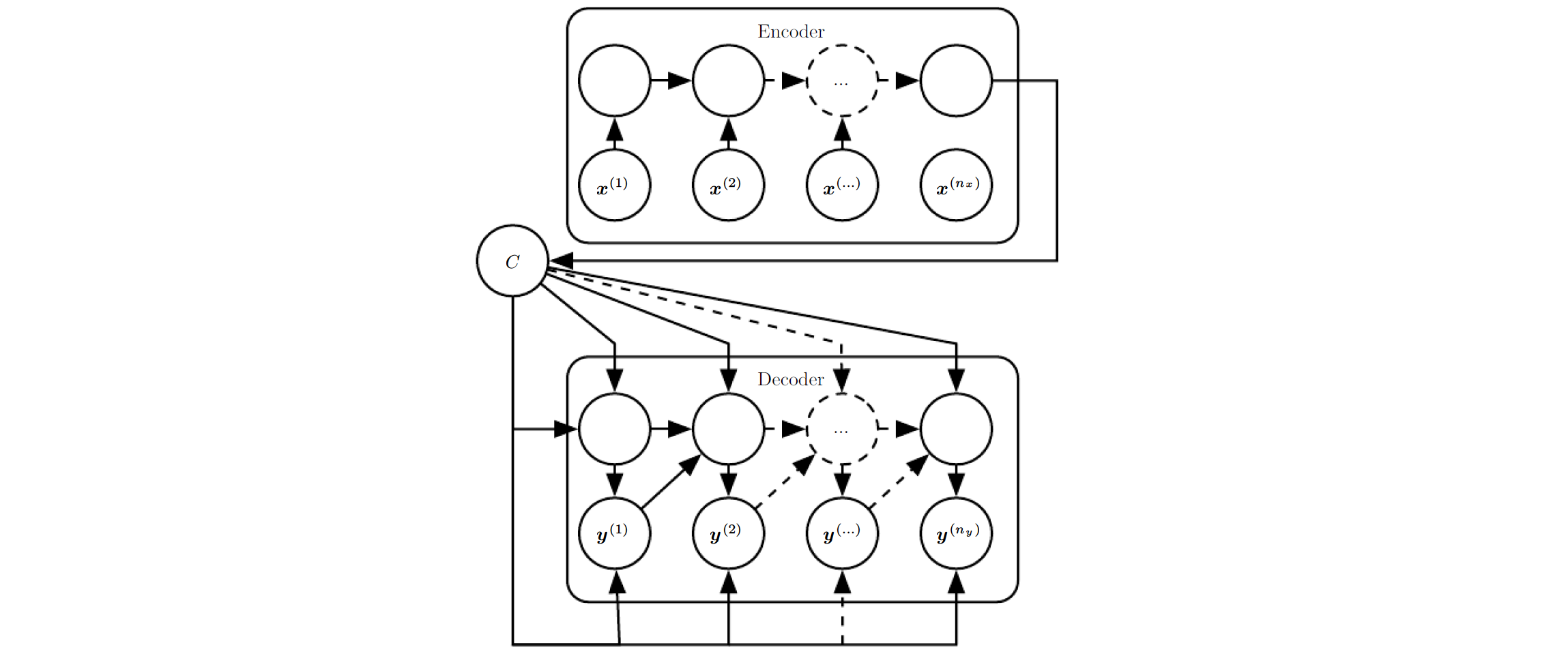(1) 编码-解码结构

编码 (encoder/reader/input) RNN 处理输入序列，输出上下文 C $C$ (通常为最后隐含状态的简单函数)。
解码 (decoder/writer/output) RNN 输入单个定长的向量 (见”向量输入”部分的x$x$)，输出语句 Y=(y(1),...,y(ny)) $Y=(y^{(1)},...,y^{(n_y)})$

该结构的创新处为 nxny $n_x和n_y$可变，而之前所述的结构有约束 nx=ny=τ $n_x=n_y=\tau$

编码 RNN 的最后的状态 hnx $h_{nx}的$输出 C $C$为解码 RNN 的输入。如果C$C$为向量，解码 RNN 仅仅是1个向量到序列的 RNN (见”向量输入”部分)。

编码器的隐含单元数目与解码器的隐含单元数目可以不相等。

2015 年 Bahdanau 等人在机器翻译的上下文中观察到，编码 RNN 的上下文输出 C $C$的维度太小会难以合适地概括长序列，因此它们把C$C$从1个定长向量改为变长序列。另外，引入学习关联序列 C $C$元素至输出序列元素的注意机制。

(2) 序列到序列的结构

联合训练两个 RNN 来最大化训练集中所有xy$x-y$序列对的 logP(y1,...,yny|x1,...,xnx) $log\phantom{1}P(y^{1},...,y^{n_y}|x^{1},...,x^{n_x})$均值。

5. 深度循环网络

大多数 RNN 计算分解为参数与相关变换的3步：

• 从输入到隐含状态；
• 从上1个隐含状态到下1个隐含状态；
• 从隐含状态到输出。

每部分关联1个权重矩阵。换句话说，展开网络时，每部分的矩阵对应1个浅变换。深 MLP 中的单层变换称为浅变换。典型的浅变换用1个学得的仿射变换 (紧随1个固定的非线性) 表示。

实验证据强烈建议对浅变换引入深度，一致认为需要足够的深度来满足映射要求。

2013 年 Graves 等人首次显示分解 RNN 状态进多层的巨大收益。下图 (a) 中分层的底层可视为转换原输入成隐含状态更高层的更合适的表示。
2014 年 Pascanu 等人让上述每部分有1个 MLP，如下图 (b)。根据对每部分分配足够容量来考虑整体的表示容量，但该方法添加深度后会因优化困难而可能影响训练。
总的来说，浅层结构优化更容易，添加额外的深度延长了变量从时间步 t $t$到时间步t+1$t+1$的最短路径。比如，状态间用1个单隐含层的 MLP，则与常规 RNN 相比，任意两个不同时间步间变量的最短路径变为两倍。
然而，跳过隐含单元到隐含单元路径上的连接可缓解路径延长问题，见下图 (c)。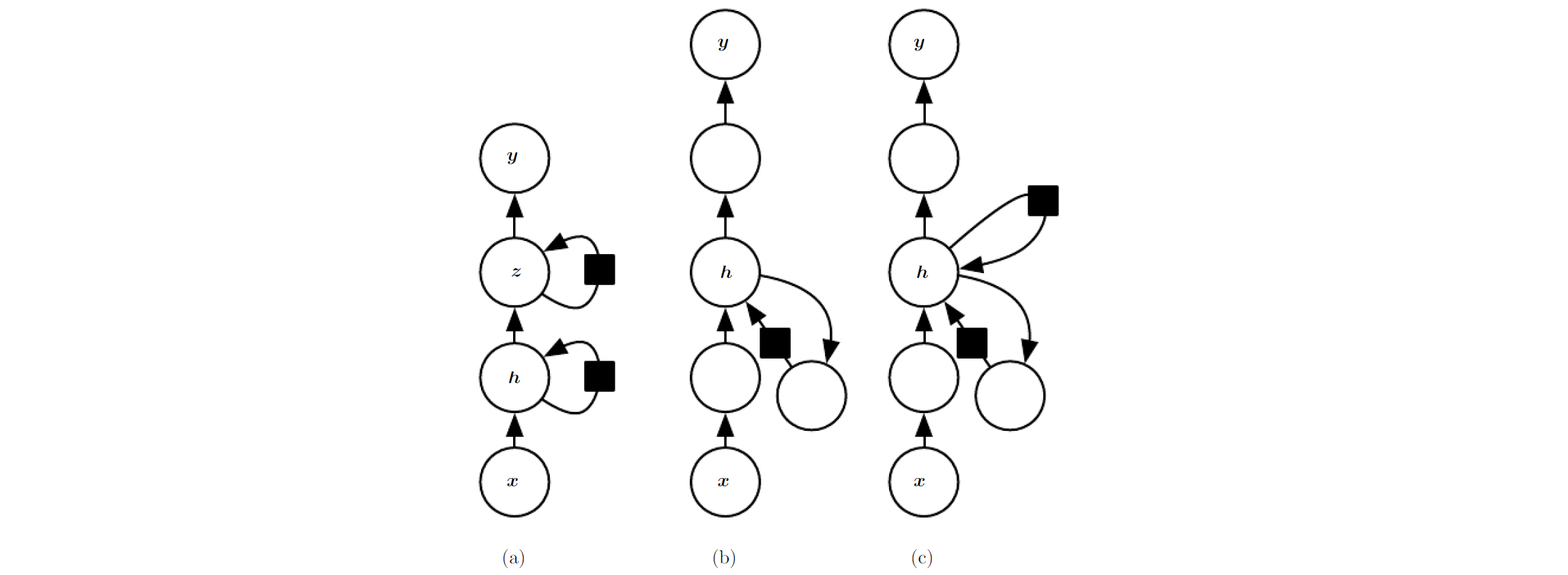与图 (b) 相比，图 (c) 的隐含单元到隐含单元的路径上，多出了1个连接与 MLP 的单隐含层并联

6. 递归网络 (Recursive Neural Networks)

递归网络是循环网络的另1种泛化，但计算图的结构为1个深层树，而不是 RNN 的链式结构。

1990 年 Pollack 提出递归网络。在 NLP 和计算机视觉方面，递归网络成功用于处理送入神经网络的数据结构

对序列长度为 τ $\tau$的循环网络，递归网络的深度 (构成非线性操作的数目) 从 τ $\tau$大幅减少至 O(logτ) $O(log\tau)$，有助于处理长期依赖 (long-term dependencies)。最好的树结构的设计问题尚未解决。1种选择为采用独立于数据的树结构 (如平衡二叉树)。某些领域中，外在方法能表现出合适的树结构。比如处理自然语句时，递归网络的树结构可固定为由自然语言解析器提供的语句解析树。学习器根据给定输入发现和推断出的树结构是理想的树结构。

递归网络的变种很多。1997 和 1998 年 Frasconi 等人用数据关联树结构，且用输入和输出关联树的节点。每个节点的计算不必是传统的人工神经计算 (所有输入的仿射变换，紧随1个单调的非线性)。比如，2013 年 Socher 发现建模概念 (连续向量表示) 间的关系时张量操作和双线性形式有用。

7. 长期依赖的挑战

循环网络中，学习长期依赖的基本问题为许多层上传播的梯度往往会消失 (常见) 或爆炸 (少见，优化伤害大)。即使参数稳定 (可保存记忆，且梯度不爆炸)，与短期作用相比，长期作用的权重指数衰减，依然难以解决问题。其它方法用更深的处理 (Hochreiter, 1991; Bengio 等, 1994; Pascanu 等, 2013)。

循环网络的多个时间步相同函数组合产生了极强的非线性。

循环网络的组成函数类似矩阵乘法。循环关系可表示为1个去掉非线性激活函数和输入 x $x$的简单循环网络：

h(t)=WTh(t1)

循环关系本质上用次方描述，可简化为：

h(t)=(Wt)Th(0)

W $W$可用正交阵Q$Q$分解为：
W=QΛQT

则该循环可简化为：
h(t)=((QΛQT)t)Th(0)=(QΛQTQΛQT...QΛQT)Th(0)=(QΛΛ...ΛQT)Th(0)=(QΛtQT)Th(0)=QTΛtQh(0)

特征值提高为它的 t $t$次方后，导致小于1的特征值衰减至0，大于0的特征值爆炸。任何h^{(0)}中的非最大特征值部分都会被丢弃。

循环网络中该问题尤为明显。假设权重ω$\omega$为标量，权重自乘很多次后会根据 ω $\omega$的幅值消失或爆炸。然而，对每个时间步权重 w(t) $w^{(t)}$不同的非循环网络不成立。如果初始状态给定为1，则 t $t$时刻的状态为tw(t)$\prod_tw^{(t)}$。假设 w(t) $w^{(t)}$从均值为 0 $0$，方差为v$v$的分布 (独立同分布) 中随机产生。则该乘积的方差为 O(vn) $O(v^{n})$。为获得理想的方差 v $v^*$，选择每个时间步的权重为 v=vn $v=\sqrt[n]{v^*}$。因此，小心选择缩放的很深前向网络可以避免梯度消失或爆炸问题。

有人希望通过保留梯度在不消失和不爆炸的参数空间区域内，不幸的是，为保存对小扰动鲁棒的区域，RNN 一定会进入梯度消失的参数空间区域。具体地，长期作用的梯度指数级地小于短期作用的梯度。这并不意味着梯度不可学，只是要花很长的时间去学长期作用，因为短期作用出现的波动常会隐藏长期作用的信号。1994 年 Bengio 等人实验表明扩大捕获的依赖范围会使基于梯度的优化难度加大，对长度为10或20的序列，用 SGD 的 传统 RNN 训练权重的成功率快速减为0。

8. 回响状态网络 (Echo State Networks)

h(t1) $h^{(t-1)}$ h(t) $h^{(t)}$的循环权重与从 x(t) $x^{(t)}$ h(t) $h^{(t)}$的输入权重为循环网络中最难学的参数。避免该问题的1种方法为让隐含单元捕获过去输入的历史，仅学习输出权重。该想法被称为回响状态网络 (ESN) 和 液态机 (liquid state machine)。与前者相比，后者用尖峰神经元 (二值输出) 替换连续值的隐含单元。两者都属于储备池计算 (reservoir computing)，储备时序特征的隐含单元可捕捉不同方面的输入历史。

储备池计算循环网络与核机器相似：映射1个任意长度的序列 (截止至时间步 t $t$的输入历史) 至1个定长的向量 (循环状态h^{(t)})，可以用线性预测器 (典型地，线性回归)。训练标准可设计为输出权重的凸函数。比如，从隐含单元到输出的映射用线性回归，且训练标准为均方差，那么该标准为凸函数且可用简单的学习算法求解。

因此，如何设置输入和循环权重使循环网络的状态能够表示1套丰富的输入历史。存储池计算给出的答案为视循环网络为1个动态系统，且设置输入和循环权重使动态系统接近稳定。

使状态至状态的转换函数的 Jacobian 特征值接近1。(?) 1个循环网络的重要特征是 Jacobians J(t)=s(t)s(t1)$J^{(t)}=\frac{\partial s^{(t)}}{\partial s^{(t-1)}}$的特征值谱。 J(t) $J^{(t)}$的谱半径定义为其特征值绝对值的最大值。

为理解谱半径的影响，考虑 t $t$不变时的 Jacobian 矩阵 J$J$ 的反向传播 (如当网络纯线性时)。假设 J $J$为对应特征λ$\lambda$的1个特征向量 v $v$。用梯度向量g$g$开始，1步反传后得到 Jg $Jg$ n $n$步反传得到J(n)g$J^{(n)}g$。换为 g $g$的扰动版本g+δv$g+\delta v$开始反传， n $n$步后得到J(n)(g+δv)$J^{(n)}(g+\delta v)$。所以，从 g+δv $g+\delta v$反传 n $n$步后多发散J(n)δv$J^{(n)}\delta v$。如果 v $v$选为特征值为λ$\lambda$ J $J$的单位特征向量，Jacobian$Jacobian$ 的乘法仅在每步乘以1个常数。两个反传的差值为 δ|λ|(n) $\delta |\lambda|^{(n)}$ v $v$对应λ$\lambda$的最大值时，该扰动得到的差值最大。

λ>1 $\lambda >1$时， δ|λ(n)| $\delta |\lambda^{(n)}|$指数增大；当 λ<1 $\lambda <1$时， δ|λ(n)| $\delta |\lambda^{(n)}|$指数减小。

该例假定每步的 Jacobian 相同，对应1个线性的循环网络。引入非线性时，非线性的导数经过许多时间步后将接近0，避免大的谱半径引起的爆炸。

用重复的矩阵乘法的反传的一切，同样适用于1个线性网络的正向传播，状态 h(t+1)=h(t)TW $h^{(t+1)}={h^{(t)}}^TW$

当线性映射 WT $W^T$总按 L2 $L^2$模缩小 h $h$，那么该映射是收缩的，每个时间步后都会变小一点。因此，当我们用有限精度来保存状态向量时，会使网络容易遗忘历史信息。

Jacobian 矩阵告诉我们h(t)$h^{(t)}$正向迭代或 h(t+1) $h^{(t+1)}$反向迭代时的微小变化。 W $W$J$J$不必为对称阵 (实数方阵)，所以它们可以有复数特征值和特征向量，虚部对应潜在的震荡行为 (迭代使用相同的 Jacobian)。尽管 h(t) $h^{(t)}$或反传中 ht $h^{t}$的小变化为实值，但可用复数形式表示。迭代应用 Jacobian 矩阵时，复数绝对值大于1时的特征值对应指数增长，否则缩小。

带非线性的 Jacobian 在每个时间步都可无约束地变化，因此具有更复杂的动态特性。然而，1个起始的小差异，若干时间步后仍然会变为大差异。 与纯线性相比，非线性网络用诸如 tanh $tanh$这样压扁的非线性导致循环的动态特性有界。注意到，即使正向传播的动态特性有界，反向传播可能会保持动态特性无界。 比如，当 tanh $tanh$单元的序列插入纯线性网络的线性区，且用谱半径大于1的权重矩阵相连。然而，所有 tanh $tanh$单元同时为线性激活点的状况很少见。

回响状态网络只是修正权重的谱半径，但因如 tanh $tanh$这样饱和非线性的稳定效果，使权重不会爆炸。

最近，设置ESN中权重的技术可用于在1个可学习的循环网络 (随时间步反传的隐含层间的循环的权重) 中初始化权重，以便学习长期依赖。其中，结合稀疏初始化模式，谱半径为1.2时效果不错。

9. 泄漏单元 (Leaky Units) 和其它多时间尺度的策略

处理长期依赖的1种方式为设计多时间尺度上操作的模型。细粒度时间尺度上操作可处理更多细节，粗粒度时间尺度上操作可更有效将遥远的历史信息转换为当前信息。所以针对既细又粗的时间尺度来设计策略：其中包含跨时间步跳过连接，“泄露单元”累积不同时间常数的信号，删除某些连接来建立细粒度时间尺度。

(1) 跨时间步跳过连接

为获取粗粒度，将遥远过去的变量与当前变量直接相连。受 1988 年 Lang 与 Hinton 在前向神经网络中插入延时的启发，1996 年 Lin 等人采用直连的方法。

梯度随时间步数的增加可能会指数地消失或爆炸。为缓解该问题， Lin 等人引入 d $d$个延时进循环连接。此时梯度指数消失可看成1个关于τd$\frac{\tau}{d}$ (而非 τ $\tau$) 的函数。

对1个指数规律衰减的量，幅值变为其 1e $\frac{1}{e}$倍所需的时间 τ $\tau$称为时间常数2 如果为 τd $\frac{\tau}{d}$，梯度会消失得更快。所以，应该改为 τd ${\tau}{d}$吧~

因延时和单步连接，梯度依然会按 τ $\tau$指数地爆炸。所以，尽管该方法不可能很好地表示所有的长期依赖，但可捕获相对更长的依赖。

(2) 泄漏单元和1个不同时间尺度谱

为使路径上的导数乘积接近1，线性自连接单元和连接权重接近1。

累积某值 v(t) $v^{(t)}$ (?) 的移动平均 μ(t) $\mu^{(t)}$：用 μ(t)αμ(t1)+(1α)v(t) $\mu^{(t)}\leftarrow \alpha \mu^{(t-1)}+(1-\alpha)v^{(t)}$更新， α $\alpha$为连接 μ(t1) $\mu^{(t-1)}$ μ(t) $\mu^{(t)}$的参数。当 α $\alpha$接近1时，移动平均可记住过去的信息很长一段时间；当 α $\alpha$接近0时，过去信息被迅速排除。线性自连接的隐含单元类似于移动平均，被称为泄露单元

跳过 d $d$个时间步的连接可确保单元总能学习到d$d$个时间步前的影响。另1种方式为用1个接近1的权重来线性自连接。线性自连接方法通过调整实数值 α $\alpha$ (而非调整整型跳过的长度)，效果更平滑和灵活。

用泄漏单元设置时间常数有两种基本策略。一种为手动固定值，比如，初始时刻从某分布中采样1次值。另一种为将时间常数设置为自由参数，然后学习它。不同时间尺度上的泄露单元有助于长期依赖。

(3) 删除连接

解决长期依赖的另1种方式为多时间尺度上组织 RNN 的状态，让信息更容易在缓慢时间尺度上长距离地流动。

与前面跳过时间步的连接不同，删除连接涉及删除时间步长度为1的连接，且替换成更长的连接。 修改后的单元只能在更长时间尺度上操作，但也可选择聚焦到短项连接上。

使循环单元在不同的时间尺度上操作的方法很多。1种为令循环单元泄漏，但不同组的单元关联不同的固定时间尺度 (1992 年 Mozer 提出，2013 年 Pascanu 等人成功应用)。另1种为对不同组的单元用不同的更新频率，在不同的时间步上显式离散地更新。 这是 1996 年 Bengio 和 2014 年 Koutnik 的方法，在许多 Benchmark 数据集上效果不错。

10. 长短时记忆 (Long Short-Term Memory) 与其它门控 (gated) RNNs

实际应用中本文最有效的序列模型为门控 RNN，包括长短时记忆和基于门控循环单元的网络。

类似泄漏单元，门控 RNN 基于使随时间的路径上的导数不消失或爆炸。泄漏单元用手动选择的常数或参数作为连接权重。门控 RNNs 将连接权重泛化成每个时间步都可能改变。

泄漏单元使网络能够在很长的时间内累积信息 (如特定的特征或类型)。然而，用到累积的信息后，网络会遗忘过去的状态。比如，当子序列组成序列，且需要通过设置过去的状态为0来遗忘它。除了手动决定何时清除状态，希望网络能学习到何时去决定清除。

(1) LSTM

1997 年 Hochreiter 和 Schmidhuber 最早提出的长短时记忆的主要贡献是引入自循环来创建使信息长期流动的路径。

使自循环取决于上下文，而非固定不变。通过使自循环的权重可门控，累积的时间尺度能动态变化。此时，即使 LSTM 固定参数，累积的时间尺度可随着输入序列而改变，因为时间常数为模型的输出。LSTM 在很多应用中极为成功，如无约束手写体识别，语音识别，手写体生成，机器翻译，图像加标题和解析。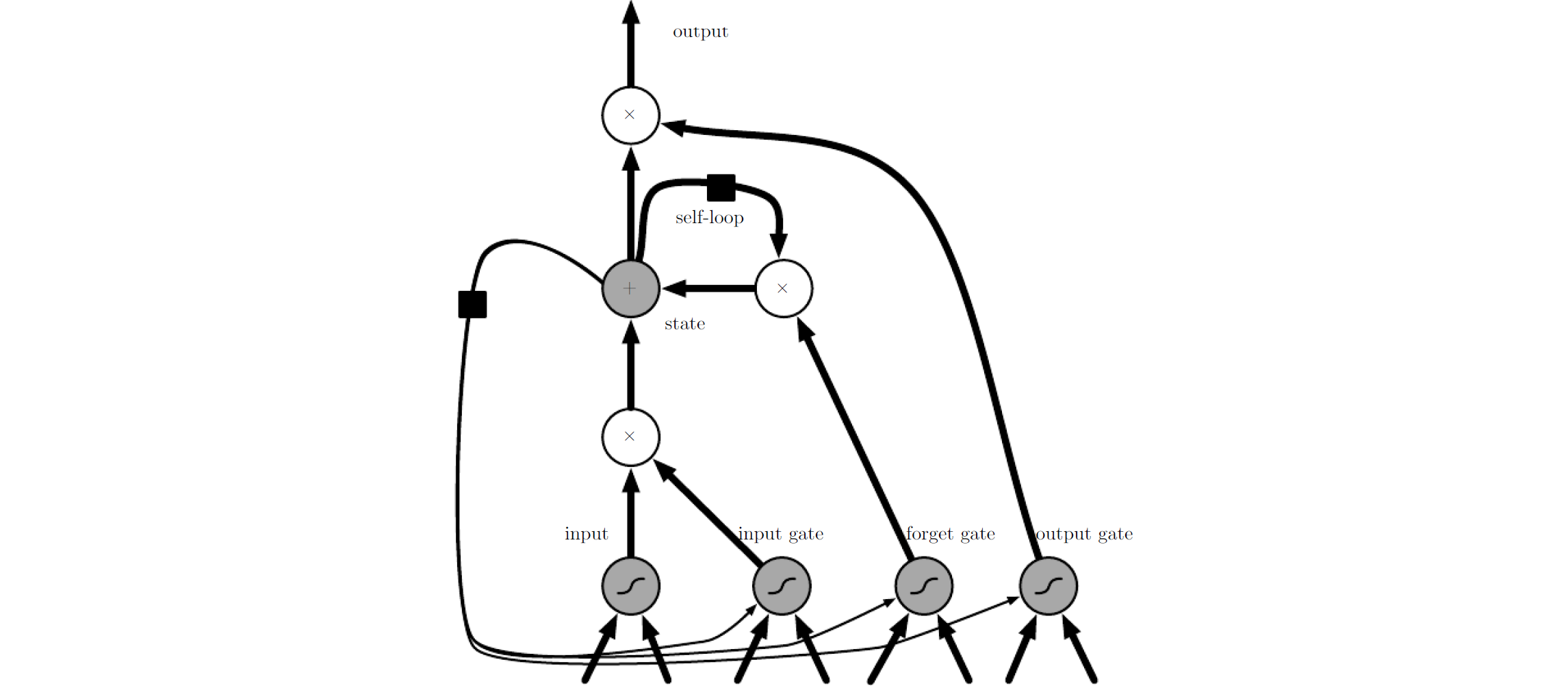LSTM 的框图见上图。LSTM 循环网络单元间彼此循环连接，来替换普通循环网络的隐含单元。用1个常规人工神经单元计算输入特征。如果 Sigmoid 门允许，输入的特征值会累加给状态。状态单元有1个由遗忘门控制的线性自循环。输出门可关闭LSTM 循环网络的输出。所有的门单元都用 Sigmoid 非线性，而输入单元可用压扁的非线性 (如 tanh)。状态单元可用作门单元的额外输入。黑色方块为1个时间步的延时。

压扁的非线性的原文为“squashing nonlinearity”。通常指各种非线性的激活函数 3，这里用 tanh 为例。

对应的前向传播方程用浅层循环网络结构给出。更深的结构也有成功的应用。每个循环单元不再是仅对输入到循环单元的仿射变换按元素应用非线性，除了 RNN 外部循环，LSTM 循环网络有“LSTM 单元”，每个单元有1个内部自循环。每个“LSTM 单元”有与普通 RNN 相同的输入和输出，但有更多的参数且有1个控制信息流的门控单元。

最重要的部分是状态单元 s(t)i $s^{(t)}_i$，具有1个与泄漏单元相似的线性自循环。但遗忘门 (forget gate) 单元 f(t)i $f_i^{(t)}$ (时间步 t $t$的单元i$i$) 用1个 Sigmoid 单元设置权重至 0 $0$~1$1$之间，来控制自循环的权重 (或相关时间常数)：

f(t)i=σbfi+jUfi,jx(t)j+jWfi,jh(t1)j

其中， x(t) $x^{(t)}$为当前输入向量。 h(t) $h^{(t)}$为当前隐含单元向量，包含所有 LSTM 单元的输出。且 bf,Uf,Wf $b^f,U^f,W^f$分别为遗忘门的偏置，输入权重和循环权重。

用条件自循环权重 f(t)i $f_i^{(t)}来更新$LSTM 单元的内部状态。

s(t)i=f(t)is(t1)i+g(t)iσbi+jUi,jx(t)j+jWi,jh(t1)j

其中， b,U,W $b,U,W$分别为LSTM 单元的偏置，输入权重和循环权重。外部输入门 (external input gate) 单元 g(t)i $g^{(t)}_i$与遗忘门单元计算类似，但有自己的参数：
g(t)i=σbgi+jUgi,jx(t)j+jWgi,jh(t1)j

输出门 (output gate) 单元 q(t)i $q_i^{(t)}$可关闭 LSTM 单元的输出 h(t)i $h_i^{(t)}$
q(t)i=σboi+jUoi,jx(t)j+jWoi,jh(t1)jh(t)i=tanh(s(t)i)q(t)i

其中，参数 bo,Uo,Wo $b^o,U^o,W^o$分别为输出门的偏置，输入权重和循环权重。LSTM 的变种中，选择把单元状态 s(t)i $s_i^{(t)}$送入第 i $i$个单元的3个门作为额外的输入 (带权重)。

有上标f$f$的参数属于遗忘门，有上标 g $g$的参数属于外部输入门，有上标o$o$的参数属于输出门。

与简单的循环结构相比，LSTM 网络在测试长期依赖能力的合成数据集和挑战性任务处理任务上可学习到更长期的依赖。

(2) 其它门控 RNN

LSTM 哪一部分是必要的？还能设计出其它成功的结构，使网络能动态控制时间尺度和遗忘不同单元的行为?

近期门控 RNN 上的工作，网络单元为门控循环单元或 GRUs。与 LSTM 不同之处为仅有1个门单元同时控制遗忘因子和决定是否更新状态单元。更新方程如下：

h(t)i=u(t1)ih(t1)i+(1u(t1)i)σbi+jUi,jx(t1)j+jWi,jr(t1)jh(t1)j

其中， u $u$为更新门，r$r$为复位门。它们的值定义为：
u(t)i=σbui+jUui,jx(t)j+jWui,jh